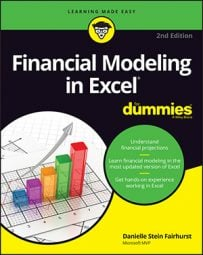##### Financial Modeling in Excel For DummiesIf you need to report sales in your financial model, use SUMIF. SUMIF is similar to COUNTIF, but it sums rather than counts the values of cells in a range that meet given criteria. Following on from the last example, let’s say you want to know how much (in terms of dollar value) in sales were made in each region. To solve this problem, follow these steps:
1. In cell F1, type “No. Sales” and format if necessary.
2. In cell F2, type =SUMIF( and press Ctrl+A.

The Function Arguments dialog box appears.

3. In the Range field, enter the items you’re adding together (B2:B22), and then press F4.
4. In the Criteria field, enter the criteria you’re looking for in that range (E2).

You don’t press the F4 key here, because you want to copy it down the column.

5. In the Sum_range field, enter the numbers you want to sum together (C2:C22), and then press F4.

Check out what this should look like.

6. Click OK.

The resulting formula will be =SUMIF(\$B\$2:\$B\$22,E2,\$C\$2:\$C\$22) with the calculated value of \$99,310.

7. Copy the formula down the column.
8. Click cell G6, use the shortcut Alt+=, and press Enter to add the sum total.

The calculated value is \$384,805.

9. Format as necessary.
10. In cell G7, enter the formula =SUM(C2:C22)-G6 to make sure the totals are the same.
11. Format the zero to a dash by clicking the comma button in the Number section of the Home tab.
12. Check your numbers against this example.

You’ve now got a summary report at the bottom, showing you how much you’ve sold in terms of number and dollar value.